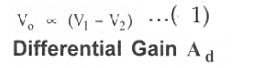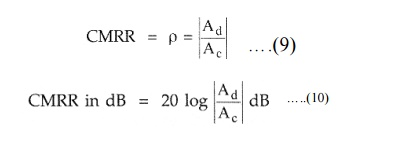Home | | Electronic Circuits I | Introduction of Differential Amplifier

# Introduction of Differential Amplifier

A device which accepts an input signal and produces an output signal proportional to the input, is called an amplifier. An amplifier which amplifies the difference between.the two input signals is called differential amplifier.

Introduction of Differential Amplifier

A device which accepts an input signal and produces an output signal proportional to the input, is called an amplifier. An amplifier which amplifies the difference between.the two input signals is called differential amplifier. The differential amplifier configuration is used in variety of analog circuits. The.differential amplifier is an essential and basic building block in modern IC amplifier .The Integrated Circuit (IC) technology is well known now a days, due to which the design of complex circuits become very simple. The IC version of operational amplifier is inexpensive, takes up less space and consumes less power. The.differential amplifier is the basic building block of such IC operational amplifier.

Basics of Differential Amplifier

The Differential Amplifier amplifies the difference between two input voltage signal. Hence it is also called as difference amplifier.

Consider an ideal differential amplifier shown in the Fig. AV1 and V2 are the two input signals while Vo is the output. Each signal is measured with respect to the ground.

In an ideal differential amplifier, the output voltage Vo is proportional to the difference between the two input signals. Hence we can write,From Equation 1 we can write,where AD is the constant of proportionality. The AD is the gain with which differential amplifier amplifies the difference between two input signals. Thus it is called differential gain of the differential amplifier.

The difference between the two inputs (V1 - V2) is generally called difference voltage and denoted as Vd.Hence the differential gain can be expressed as,Generally the differential gain is expressed in its decibel (dB) value as,Common Mode Gain Ac

If we apply two input voltages which are equal in all the respects to the differential amplifier i.e. V1  = Vz then ideally the output voltage Vo = (V1 - V2) Ad, must be zero.But the output voltage of the practical differential amplifier not only depends on thedifference voltage but also depends on the average common level of the two inputs.

Such an average level of the two input signals is called common mode signal denoted as VCPractically, the differential amplifier produces the output voltage proportional to such common mode signal, also. The gain with which it amplifies the common mode signal to produce the output is called common mode gain of the differential amplifier AC.\Thus there exists some finite output for V1  = V2  due to such common mode gain AC, in case of practical differential amplifiers.

So the total output of any differential amplifier can be expressed as,For an ideal differential amplifier, the differential gain Ad, must be infinite while the common mode gain must be zero.

But due to mismatch in the internal circuitry, there is some output available for

V1 = V2 and gain AC is not practically zero. The value of such common mode gain AC very small while the value of the differential gain Ad is always very large.

Common Mode Rejection Ratio (CMRR)

When the same voltage is applied to both the inputs, the differential amplifier is said to be operated in a common mode configuration. Many disturbance signals, noise signal appear as a common input signal to both the input terminals of the differential amplifier. Such a common signal should be rejected by the differential amplifier. The ability of a differential amplifier to reject a common mode signal is expressed by a ratio called common mode rejection ratio denoted as CMRR. It is defined as the ratio of the differential voltage gain Ad to common mode voltage gain ACStudy Material, Lecturing Notes, Assignment, Reference, Wiki description explanation, brief detail
Electronic Circuits : BJT Amplifiers : Introduction of Differential Amplifier |